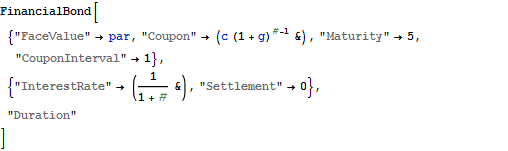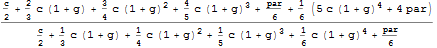Neu in Mathematica 8 – Financial Engineering

Financial Engineering

For a long time now, Mathematica has been especially focussed on financial mathematical functions, allowing for the successes in this enormously significant core fiel of economy. Via "curated data" in Mathematica, it is possible to retrieve financial data online from the servers at Wolfram Research and analyse them. With Mathematica 8, Wolfram Research adds special financial mathematical instruments in form of charts.
• A large number of available bonds and derivatives
• Time-related calculations of annuities, interest rates, cashflows
• Implied volatility and computation of Greeks

Tight Integration of financial functions

Compute the value of an option, based on the latest price of the stock and continuous-compounding equivalent interest rate.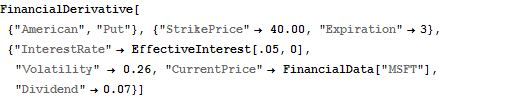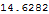Control Numerical Evaluation

Compute the critical value surface of an American put option using a custom number of grid points.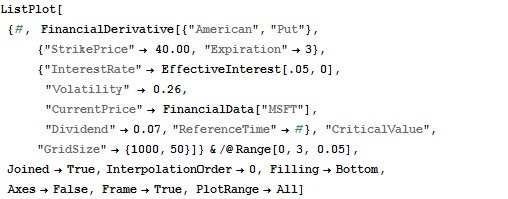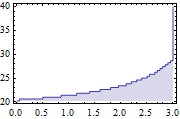Exotic Contracts

Handling for a number of exotic contracts, for example, perpetual lookback contracts.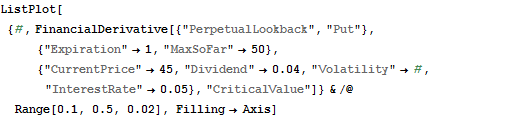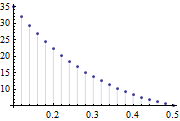Computation of Greeks

Compute the sensitivities to various parameters.

To show Mathematica code, please click "plus".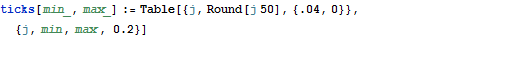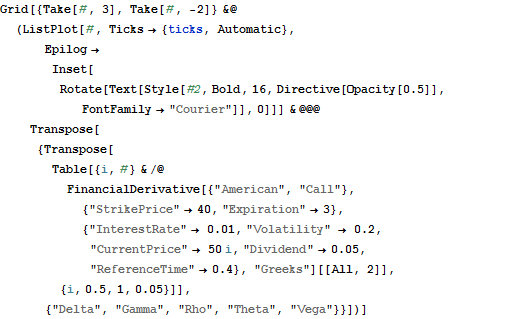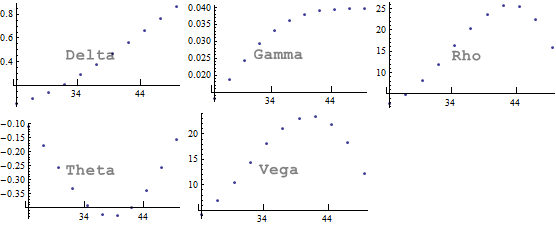Applications

Construct a synthetic short position on a stock by using the capabilities of "FinancialDerivative".

To show Mathematica code, please click "plus".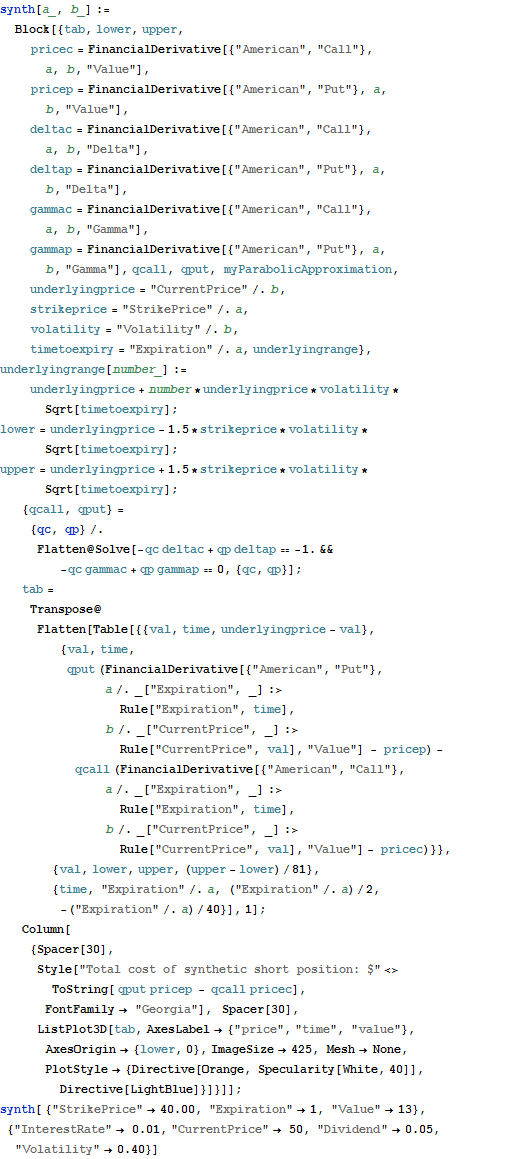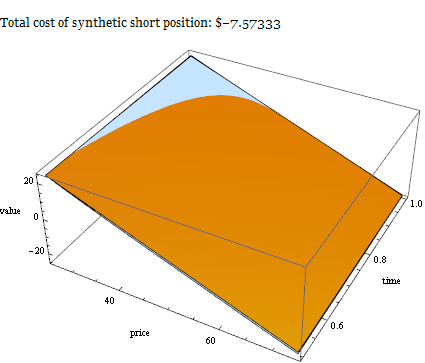Input Symbolic Parameters

Many financial functions take symbolic parameters and can return expressions in symbolic form.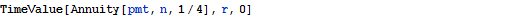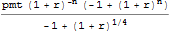Plot Values as a Function of any Variable

The symbolic structure of many financial functions allows for easy visualization.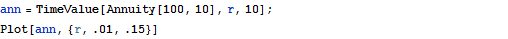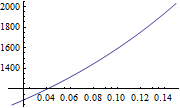Find Solutions for Specified Variables

Solve, FindRoot, and other functions can be used to find solutions for symbolic variables.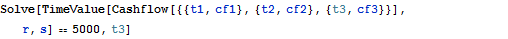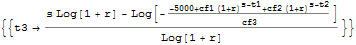Assets with Growing Payments

"Annuity", "AnnuityDue", and "FinancialBond" can take payment arguments in a functional form to specify payment structures that vary with time.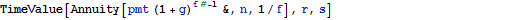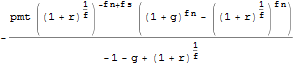Combine Interest Rate and Payment Processes

FinancialBond and Annuity allow for the calculation of values and other measures under the combination of functional interest rate and payment processes.